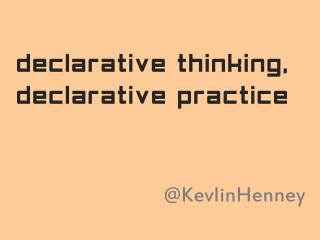DownloadDownload PresentationDeclarative Thinking, Declarative Practice

# Declarative Thinking, Declarative Practice

Télécharger la présentation## Declarative Thinking, Declarative Practice

- - - - - - - - - - - - - - - - - - - - - - - - - - - E N D - - - - - - - - - - - - - - - - - - - - - - - - - - -
##### Presentation Transcript

1. declarative thinking, declarative practice @KevlinHenney

2. Computer Science in the 1960s to 80s spent a lot of effort making languages which were as powerful as possible. Nowadays we have to appreciate the reasons for picking not the most powerful solution but the least powerful. Tim Berners-Lee https://www.w3.org/DesignIssues/Principles.html

3. The reason for this is that the less powerful the language, the more you can do with the data stored in that language. If you write it in a simple declarative form, anyone can write a program to analyze it in many ways. Tim Berners-Lee https://www.w3.org/DesignIssues/Principles.html

4. The makefile language is similar to declarative programming. This class of language, in which necessary end conditions are described but the order in which actions are to be taken is not important, is sometimes confusing to programmers used to imperative programming. http://en.wikipedia.org/wiki/Make_(software)

5. Representation Is the Essence of Programming

6. Show me your flowcharts and conceal your tables, and I shall continue to be mystified. Show me your tables, and I won’t usually need your flowcharts; they’ll be obvious.

7. Excel is the world's most popular functional language. Simon Peyton-Jones

8. try { Integer.parseInt(time.substring(0, 2)); } catch (Exception x) { return false; } if (Integer.parseInt(time.substring(0, 2)) > 12) { return false; } ... if (!time.substring(9, 11).equals("AM") & !time.substring(9, 11).equals("PM")) { return false; } Burk Hufnagel "Put the Mouse Down and Step Away from the Keyboard"

9. public static boolean validateTime(String time) { return time.matches("(0[1-9]|1[0-2]):[0-5][0-9]:[0-5][0-9] ([AP]M)"); } Burk Hufnagel "Put the Mouse Down and Step Away from the Keyboard"

10. Many programming languages support programming in both functional and imperative style but the syntax and facilities of a language are typically optimised for only one of these styles, and social factors like coding conventions and libraries often force the programmer towards one of the styles. https://wiki.haskell.org/Functional_programming

11. intension, n. (Logic)  the set of characteristics or properties by which the referent or referents of a given expression is determined; the sense of an expression that determines its reference in every possible world, as opposed to its actual reference. For example, the intension of prime number may be having non-trivial integral factors, whereas its extension would be the set {2, 3, 5, 7, ...}. E J Borowski and J M Borwein Dictionary of Mathematics

12. { x2 | x   , x ≥ 1   x ≤ 100 } from where select

13. A list comprehension is a syntactic construct available in some programming languages for creating a list based on existing lists. It follows the form of the mathematical set-builder notation (set comprehension) as distinct from the use of map and filter functions. http://en.wikipedia.org/wiki/List_comprehension

14. { x2 | x   , x ≥ 1 }

15. Lazy Lazy evaluation evaluation

16. def fizzbuzz(n): result = '' if n % 3 == 0: result += 'Fizz' if n % 5 == 0: result += 'Buzz' if not result: result = str(n) return result

17. def fizzbuzz(n): if n % 15 == 0: return 'FizzBuzz' elif n % 3 == 0: return 'Fizz' elif n % 5 == 0: return 'Buzz' else: return str(n)

18. def fizzbuzz(n): return ( 'FizzBuzz' if n % 15 == 0 else 'Fizz' if n % 3 == 0 else 'Buzz' if n % 5 == 0 else str(n))

19. def fizzbuzz(n): return ( 'FizzBuzz' if n in range(0, 101, 15) else 'Fizz' if n in range(0, 101, 3) else 'Buzz' if n in range(0, 101, 5) else str(n))

20. fizzes = [''] + ([''] * 2 + ['Fizz']) * 33 + [''] buzzes = [''] + ([''] * 4 + ['Buzz']) * 20 numbers = list(map(str, range(0, 101))) def fizzbuzz(n): return fizzes[n] + buzzes[n] or numbers[n]

21. actual = [fizzbuzz(n) for n in range(1, 101)] truths = [ every result is 'Fizz', 'Buzz', 'FizzBuzz' or a decimal string, every decimal result corresponds to its ordinal position, every third result contains 'Fizz', every fifth result contains 'Buzz', every fifteenth result is 'FizzBuzz', the ordinal position of every 'Fizz' result is divisible by 3, the ordinal position of every 'Buzz' result is divisible by 5, the ordinal position of every 'FizzBuzz' result is divisible by 15 ] all(truths)

22. actual = [fizzbuzz(n) for n in range(1, 101)] truths = [ all(a in {'Fizz', 'Buzz', 'FizzBuzz'} or a.isdecimal() for a in actual), all(int(a) == n for n, a in enumerate(actual, 1) if a.isdecimal()), all('Fizz' in a for a in actual[2::3]), all('Buzz' in a for a in actual[4::5]), all(a == 'FizzBuzz' for a in actual[14::15]), all(n % 3 == 0 for n, a in enumerate(actual, 1) if a == 'Fizz'), all(n % 5 == 0 for n, a in enumerate(actual, 1) if a == 'Buzz'), all(n % 15 == 0 for n, a in enumerate(actual, 1) if a == 'FizzBuzz') ] all(truths)